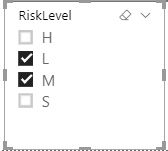cancel
Showing results for
Did you mean:Frequent Visitor

## Multiple Selection for Slicer/Parameter

Hi -

I have a time based measure that calculates a "Risk" metric based on several other attributes which change over time.I'd like to add a slicer which allows multi-select like the one below where I can filter down on the Risk metrics.However, I'm having trouble figuring out how to move forward. It seems like after searching the forum, there may be some combination of using VALUES and IN but I can't quite figure it out.  I also saw someone use ISCROSSFILTERED and then filter when it's true but still running into issues with it.  Thanks!

1 ACCEPTED SOLUTIONCommunity Support

HI @facilitydax ,

Did your slicer use the same source field as your snapshot? If this is a case, you can direct use values(Table[Risk]) as a filter condition to filter calculation records.

``````Measure =
CALCULATE ( formula, VALUES ( Table[Risk] ) )
``````

If your slicer uses an unrelated table column as the source, you can loop the whole table to compare it with selected items.

``````Measure =
CALCULATE (
formula,
FILTER ( ALLSELECTED ( Table ), Table[Risk] IN VALUES ( selector[Risk] ) )
)

``````

Regards,

Xiaoxin Sheng

Community Support Team _ Xiaoxin
If this post helps, please consider accept as solution to help other members find it more quickly.Community Support

HI @facilitydax ,

Did your slicer use the same source field as your snapshot? If this is a case, you can direct use values(Table[Risk]) as a filter condition to filter calculation records.

``````Measure =
CALCULATE ( formula, VALUES ( Table[Risk] ) )
``````

If your slicer uses an unrelated table column as the source, you can loop the whole table to compare it with selected items.

``````Measure =
CALCULATE (
formula,
FILTER ( ALLSELECTED ( Table ), Table[Risk] IN VALUES ( selector[Risk] ) )
)

``````

Regards,

Xiaoxin Sheng

Community Support Team _ Xiaoxin
If this post helps, please consider accept as solution to help other members find it more quickly.